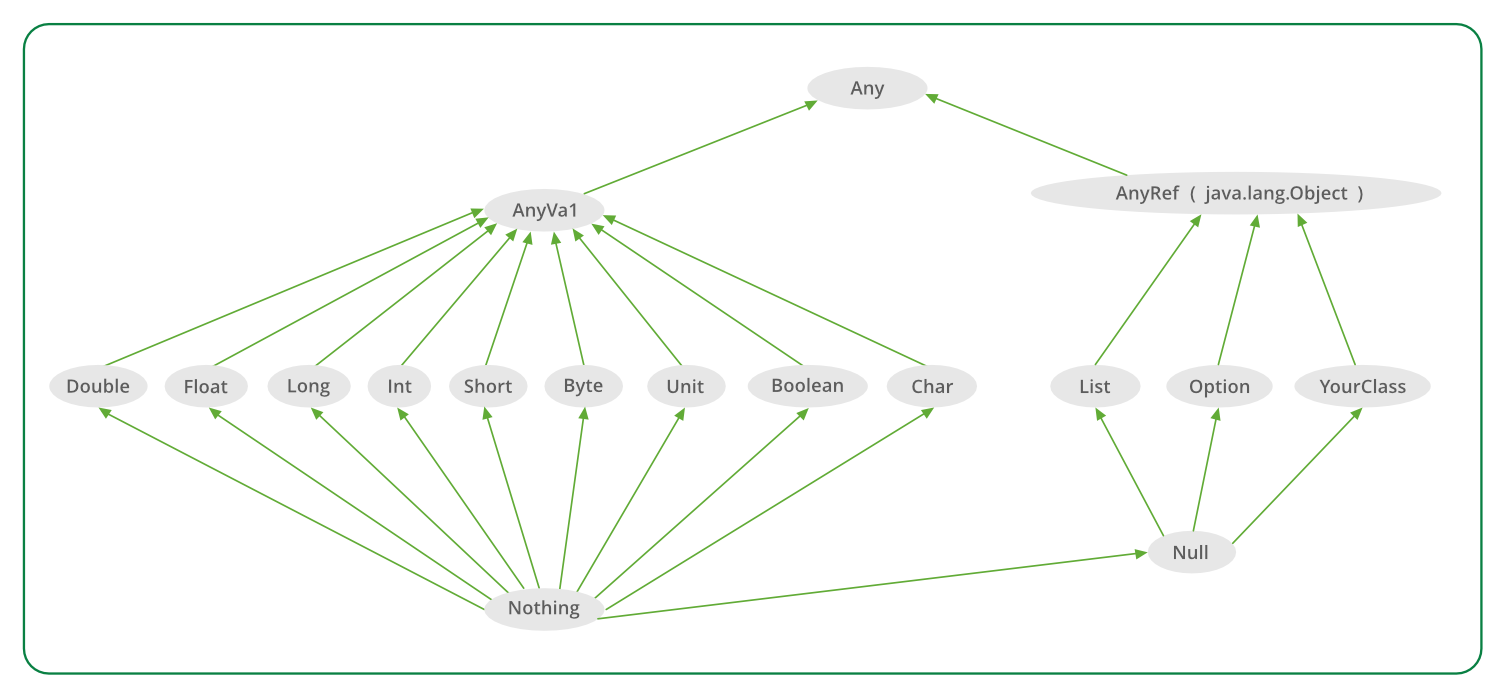# Scala Type Hierarchy

• Last Updated : 01 Apr, 2019

There are no primitive types in Scala(unlike java). All data types in Scala are objects that have methods to operate on their data. All of Scala’s types exist as part of a type hierarchy.

Every class that we define in Scala will also belong to this hierarchy automatically.Any is the superclass of all classes, also called the top class. It defines certain universal methods such as equals, hashCode, and toString. Any has two direct subclasses:

• AnyVal
• AnyRef

.
Example:

 `// Scala program of Scala Type hierarchy `` ` `// Creating object``object` `Geeks ``{`` ` `    ``// Main method``    ``def` `main(args``:` `Array[String]) ``    ``{``        ``val` `list``:` `List[Any] ``=` `List(``                ``false``,``                ``66677``,``                ``732``, ``                ``'a'``, ``                ``"abs"``                    ``)`` ` `        ``list.foreach(element ``=>` `println(element))``    ``}``}`

Output:

```false
66677
732
a
abs
```

AnyVal represents value classes. All value classes are predefined; they correspond to the primitive types of Java-like languages. There are nine predefined value types and they are non-null able: Double, Float, Long, Int, Short, Byte, Char, Unit, and Boolean. Scala has both numeric (e.g., Int and Double) and non-numeric types (e.g., String) that can be used to define values and variables. Boolean variables can only be true or false. Char literals are written with single-quotes.

Example:

 `// Scala program of Scala Type hierarchy``// Using AnyVal. `` ` `// Creating Object``object` `Geeks ``{`` ` `    ``// Main method``    ``def` `main(args``:` `Array[String]) ``    ``{``        ``val` `list``:` `List[AnyVal] ``=` `List(``            ``333``, ``true``, ``false``            ``)`` ` `        ``list.foreach(element ``=>` `println(element))``    ``}``}`

Output:

```333
true
false
```

AnyRef represents reference classes. All non-value types are defined as reference types. User-defined classes define reference types by default; i.e. they always (indirectly) subclass scala.AnyRef. scala.AnyRef in java programming corresponds to java.lang.Object.

Example:

 `// Scala program of Scala Type hierarchy ``// Using AnyRef`` ` `// Creating object``object` `Geeks``{`` ` `    ``// Main method``    ``def` `main(args``:` `Array[String])``    ``{``        ``val` `list``:` `List[AnyRef] ``=` `List(``        ``"GFG"``, ``"GEEKSFORGEEKS"``            ``)`` ` `        ``list.foreach(element ``=>` `println(element))``    ``}``}`

Output:

```GFG
GEEKSFORGEEKS
```

Nothing and Null:
Nothing is a subclassify of all value types, it is also called the bottom type. Type Nothing That has no value. we can use Nothing to signal non-termination such as a thrown exception, program exit, or an infinite loop .

Null is a subclassify of all reference types. the keyword literal null can identify a single value. Null is provided mostly for interoperability with other JVM languages.

My Personal Notes arrow_drop_up